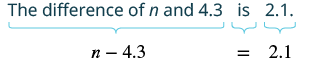## Translating Statements to Equations Containing Decimals

### Learning Outcomes

• Translate statements containing decimals into algebraic equations and solve

Now that we have solved equations with decimals, we are ready to translate word sentences to equations and solve. Remember to look for words and phrases that indicate the operations to use.

### example

Translate and solve: The difference of $n$ and $4.3$ is $2.1$.

Solution

 Translate.Add $4.3$ to both sides of the equation. $n-4.3\color{red}{+ 4.3}=2.1\color{red}{+ 4.3}$ Simplify. $n=6.4$ Check: Is the difference of $n$ and $4.3$ equal to $2.1$? Let $n=6.4$ : Is the difference of $6.4$ and $4.3$ equal to $2.1$? Translate. $6.4-4.3\stackrel{?}{=}2.1$ Simplify. $2.1=2.1\quad\checkmark$

### try it

The following video contains more examples of using the language of algebra to translate a statement containing subtraction. Note that the video does not contain decimal specific examples.

### example

Translate and solve: The product of $-3.1$ and $x$ is $5.27$

### try it

The video that follows contains examples of how to use the language of algebra to translate an expression that contains multiplication. Note that the examples do not contain decimals.

### example

Translate and solve: The quotient of $p$ and $-2.4$ is $6.5$.

### try it

The video that follows gives examples of how to use the language of algebra to translate an expression that contains division. Note that the examples do not contain decimals.

### example

Translate and solve: The sum of $n$ and $2.9$ is $1.7$.

### TRY it

The video that follows gives examples of how to use the language of algebra to translate an expression that contains addition. Note that the examples do not contain decimals.

## Contribute!

Did you have an idea for improving this content? We’d love your input.